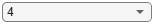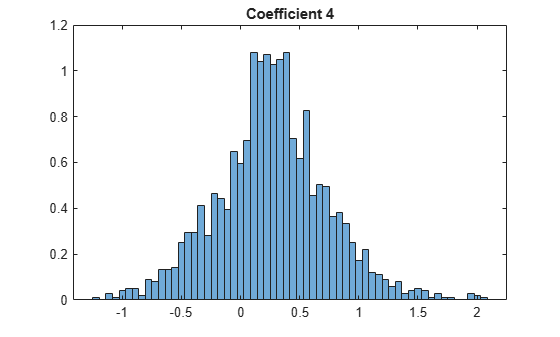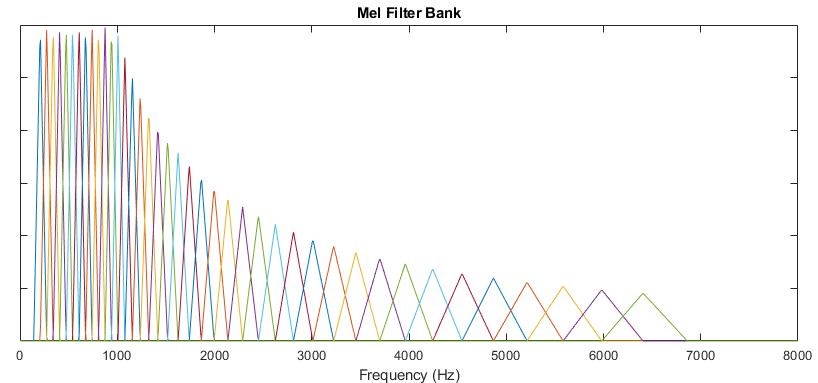# mfcc

Extract MFCC, log energy, delta, and delta-delta of audio signal

## Syntax

``coeffs = mfcc(audioIn,fs)``
``coeffs = mfcc(___,Name,Value)``
``[coeffs,delta,deltaDelta,loc] = mfcc(___)``

## Description

example

````coeffs = mfcc(audioIn,fs)` returns the mel frequency cepstral coefficients (MFCCs) for the audio input, sampled at a frequency of `fs` Hz.```
````coeffs = mfcc(___,Name,Value)` specifies options using one or more `Name,Value` pair arguments.Example: `coeffs = mfcc(audioIn,fs,'LogEnergy','Replace')` returns mel frequency cepstral coefficients for the audio input signal sampled at `fs` Hz. The first coefficient in the `coeffs` vector is replaced with the log energy value.```
````[coeffs,delta,deltaDelta,loc] = mfcc(___)` also returns the delta, delta-delta, and location of samples corresponding to each window of data.```

## Examples

collapse all

Compute the mel frequency cepstral coefficients of a speech signal using the `mfcc` function. The function returns `delta`, the change in coefficients, and `deltaDelta`, the change in delta values. The log energy value that the function computes can prepend the coefficients vector or replace the first element of the coefficients vector. This is done based on whether you set the `'LogEnergy'` argument to `'Append'` or `'Replace'`.

Read an audio signal from the `'Counting-16-44p1-mono-15secs.wav'` file using the `audioread` function. The `mfcc` function processes the entire speech data in a batch. Based on the number of input rows, the window length, and the overlap length, `mfcc` partitions the speech into 1551 frames and computes the cepstral features for each frame. Each row in the `coeffs` matrix corresponds to the log-energy value followed by the 13 mel-frequency cepstral coefficients for the corresponding frame of the speech file. The function also computes `loc`, the location of the last sample in each input frame.

```[audioIn,fs] = audioread('Counting-16-44p1-mono-15secs.wav'); [coeffs,delta,deltaDelta,loc] = mfcc(audioIn,fs);```

Read in an audio file and convert it to a frequency representation.

```[audioIn,fs] = audioread("Rainbow-16-8-mono-114secs.wav"); win = hann(1024,"periodic"); S = stft(audioIn,"Window",win,"OverlapLength",512,"Centered",false);```

To extract the mel-frequency cepstral coefficients, call `mfcc` with the frequency-domain audio. Ignore the log-energy.

`coeffs = mfcc(S,fs,"LogEnergy","Ignore");`

In many applications, MFCC observations are converted to summary statistics for use in classification tasks. Plot a probability density function for one of the mel-frequency cepstral coefficients to observe its distributions.

```nbins = 60; coefficientToAnalyze =4; histogram(coeffs(:,coefficientToAnalyze+1),nbins,"Normalization","pdf") title(sprintf("Coefficient %d",coefficientToAnalyze))```## Input Arguments

collapse all

Input signal, specified as a vector, matrix, or 3-D array.

• If `audioIn` is real, it is interpreted as a time-domain signal and must be a column vector or a matrix. Columns of the matrix are treated as independent audio channels.

• If `audioIn` is complex, it is interpreted as a frequency-domain signal. In this case, `audioIn` must be an L-by-M-by-N array, where L is the number of DFT points, M is the number of individual spectrums, and N is the number of individual channels.

Data Types: `single` | `double`
Complex Number Support: Yes

Sample rate of the input signal in Hz, specified as a positive scalar.

Data Types: `single` | `double`

### Name-Value Arguments

Specify optional comma-separated pairs of `Name,Value` arguments. `Name` is the argument name and `Value` is the corresponding value. `Name` must appear inside quotes. You can specify several name and value pair arguments in any order as `Name1,Value1,...,NameN,ValueN`.

Example: ```[coeffs,delta,deltaDelta,loc] = mfcc(audioIn,fs,'LogEnergy','Replace','DeltaWindowLength',5)``` returns mel frequency cepstral coefficients for the audio input signal sampled at `fs` Hz. The first coefficient in the `coeffs` vector is replaced with the log energy value. A set of 5 cepstral coefficients is used to compute the delta and the delta-delta values.

Window applied in time domain, specified as the comma-separated pair consisting of `'Window'` and a real vector. The number of elements in the vector must be in the range [1,`size(audioIn,1)`]. The number of elements in the vector must also be greater than `OverlapLength`.

Data Types: `single` | `double`

Number of samples overlapped between adjacent windows, specified as the comma-separated pair consisting of `'OverlapLength'` and an integer in the range [0, `numel(Window)`). If unspecified, `OverlapLength` defaults to `round(0.02*fs)`.

Data Types: `single` | `double`

Number of coefficients returned for each window of data, specified as an integer in the range [2 v], where v is the number of valid passbands.

The number of valid passbands is defined as ```sum(BandEdges <= floor(fs/2))-2```. A passband is valid if its edges fall below `fs/2`, where fs is the sample rate of the input audio signal, specified as the second argument, `fs`.

Data Types: `single` | `double`

Band edges of the filter bank in Hz, specified as a nonnegative monotonically increasing row vector in the range [0, `fs`/2]. The number of band edges must be in the range [4, 160]. The `mfcc` function designs half-overlapped triangular filters based on `BandEdges`. This means that all band edges, except for the first and last, are also center frequencies of the designed bandpass filters.

By default, `BandEdges` is a 42-element vector, which results in a 40-band filter bank that spans approximately 133 Hz to 6864 Hz. The default bands are spaced as described in .

Data Types: `single` | `double`

Number of bins used to calculate the discrete Fourier transform (DFT) of windowed input samples. The FFT length must be greater than or equal to the number of elements in the `Window`.

Data Types: `single` | `double`

Type of nonlinear rectification applied prior to the discrete cosine transform, specified as `'log'` or `'cubic-root'`.

Data Types: `char` | `string`

Number of coefficients used to calculate the delta and the delta-delta values, specified as the comma-separated pair consisting of `'DeltaWindowLength'` and an odd integer greater than two. If unspecified, `DeltaWindowLength` defaults to `9`.

Deltas are computed using the `audioDelta` function.

Data Types: `single` | `double`

Specify how the log energy is shown in the coefficients vector output, specified as:

• `'Append'` –– The function prepends the log energy to the coefficients vector. The length of the coefficients vector is 1 + `NumCoeffs`.

• `'Replace'` –– The function replaces the first coefficient with the log energy of the signal. The length of the coefficients vector is `NumCoeffs`.

• `'Ignore'` –– The object does not calculate or return the log energy.

Data Types: `char` | `string`

## Output Arguments

collapse all

Mel frequency cepstral coefficients, returned as an L-by-M matrix or an L-by-M-by-N array, where:

• L –– Number of analysis windows the audio signal is partitioned into. The input size, `Window`, and `OverlapLength` control this dimension: ```L = floor((size(audioIn,1) − numel(Window)))/(numel(Window) − OverlapLength) + 1```.

• M –– Number of coefficients returned per frame. This value is determined by `NumCoeffs` and `LogEnergy`.

When `LogEnergy` is set to:

• `'Append'` –– The function prepends the log energy value to the coefficients vector. The length of the coefficients vector is 1 + `NumCoeffs`.

• `'Replace'` –– The function replaces the first coefficient with the log energy of the signal. The length of the coefficients vector is `NumCoeffs`.

• `'Ignore'` –– The function does not calculate or return the log energy. The length of the coefficients vector is `NumCoeffs`.

• N –– Number of input channels (columns). This value is `size(audioIn,2)`.

Data Types: `single` | `double`

Change in coefficients from one frame of data to another, returned as an L-by-M matrix or an L-by-M-by-N array. The `delta` array is the same size and data type as the `coeffs` array.

Data Types: `single` | `double`

Change in `delta` values from one frame of data to another, returned as an L-by-M matrix or an L-by-M-by-N array. The `deltaDelta` array is the same size and data type as the `coeffs` and `delta` arrays.

Data Types: `single` | `double`

Location of last sample in each analysis window, returned as a column vector with the same number of rows as `coeffs`.

Data Types: `single` | `double`

## Algorithms

Mel frequency cepstrum coefficients are popular features extracted from speech signals for use in recognition tasks. In the source-filter model of speech, cepstral coefficients are understood to represent the filter (vocal tract). The vocal tract frequency response is relatively smooth, whereas the source of voiced speech can be modeled as an impulse train. As a result, the vocal tract can be estimated by the spectral envelope of a speech segment.

The motivating idea of mel frequency cepstral coefficients is to compress information about the vocal tract (smoothed spectrum) into a small number of coefficients based on an understanding of the cochlea. Although there is no hard standard for calculating the coefficients, the basic steps are outlined by the diagram.The default mel filter bank linearly spaces the first 10 triangular filters and logarithmically spaces the remaining filters.The information contained in the zeroth mel frequency cepstral coefficient is often augmented with or replaced by the log energy. The log energy calculation depends on the input domain.

If the input (audioIn) is a time-domain signal, the log energy is computed using the following equation:

`$\mathrm{log}E=\mathrm{log}\left(\text{sum}\left({x}^{2}\right)\right)$`

If the input (audioIn) is a frequency-domain signal, the log energy is computed using the following equation:

`$\mathrm{log}E=\mathrm{log}\left(\text{sum}\left({|x|}^{2}\right)/FFTLength\right)$`

## Compatibility Considerations

expand all

Behavior changed in R2020b

Behavior change in future release

 Rabiner, Lawrence R., and Ronald W. Schafer. Theory and Applications of Digital Speech Processing. Upper Saddle River, NJ: Pearson, 2010.

## SupportGet trial now